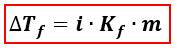Chemistry Practice Problems Freezing Point Depression Practice Problems Solution: What is Kf for water if the freezing point was low...

🤓 Based on our data, we think this question is relevant for Professor Chakraborty's class at FIU.

# Solution: What is Kf for water if the freezing point was lowered to -12°C once 30 g of Sodium Chloride was added to 150 g of water?

###### Problem

What is Kf for water if the freezing point was lowered to -12°C once 30 g of Sodium Chloride was added to 150 g of water?

###### Solution

We’re being asked to find the Kf (freezing point depression constant) of water if its freezing point decreased to -12°C when NaCl was dissolved in it. We’re going to use the Freezing Point Depression equation to calculate for Kf.∆Tf = change in freezing point = Tf pure solvent –Tf solution
Kf = freezing point depression constant
i = van' t Hoff factor of the solute = no. of ions
m = molality

Let’s first calculate the molality of the solution.View Complete Written Solution

Freezing Point Depression

Freezing Point Depression

#### Q. What is the freezing point of an aqueous solution that boils at 106°C?       Kb = 0.51°C/m and Kf = 1.86°C/m. a. −4.55 °C b. −11.8 °C c. −21.9 °C ...

Solved • Tue Jul 25 2017 18:03:48 GMT-0400 (EDT)

Freezing Point Depression

#### Q. A 5 g sample of CaI2 is completely dissolved in 22 g of water, what is the freezing point of the solution? Assume complete dissociation of the salt. K...

Solved • Fri Jun 30 2017 12:07:03 GMT-0400 (EDT)

Freezing Point Depression

#### Q. Calculate the freezing temperature of a solution made by mixing 57.267 g of CaCl2 (MW = 110.98) in 350.00 g of water. k f = 1.86 C/mA. -2.74°CB. -11.0...

Solved • Wed Apr 26 2017 11:39:22 GMT-0400 (EDT)

Freezing Point Depression

#### Q. What mass of ethanol, C2H5OH a nonelectrolyte, must be added to 10.0L of water to give a solution that freezes at -10.0°C? Assume the density of water...

Solved • Mon Mar 06 2017 12:02:41 GMT-0500 (EST)